## Pick-Place Robot

Object picking and stowing with a 6-DOF KUKA KR210 anthropomorphic robotic serial manipulator using ROS

Salman Hashmi
[email protected]

### 1. Introduction

This project originated from Udacity's Robotic arm - Pick & Place project, which, in turn is based on the Amazon Robotics Challenge sponsored by Amazon Robotics LLC.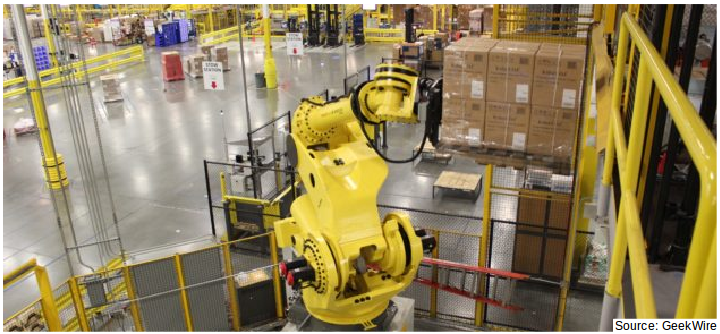Fig. 1.1  A robotic arm shelving products in an Amazon fulfillment center

##### Objective

Commercially viable automated picking and stowing in unstructured environments, like picking products off shelves and putting them into shipping boxes, still remains a difficult challenge. The goal of the ARC is to perform simplified versions of the general task of picking and stowing items on shelves. As per ARC Rules: "The Challenge combines object recognition, pose recognition, grasp planning, compliant manipulation, motion planning, task planning, task execution, and error detection and recovery".

The objective of this project is to demonstrate autonomous capability of the KR210 serial manipulator in simulation to pick and place an object in a semi-unstructured environment.

Within the context of this project, a single pick and place cycle can be divided into the following tasks:

• Identify the target object on the shelf
• Plan and perform a clean movement towards the object
• Efficiently grasp/pick the target object without disturbing other objects
• Plan and perform a clean movement towards the drop-off site
• Efficiently stow/place the object at the drop-off site
##### Relevance

The capability of picking and placing objects relies on being able to locate points of interest in a 3D environment and planning movement trajectories to those points. All robotic manipulators in industry depend on this capability.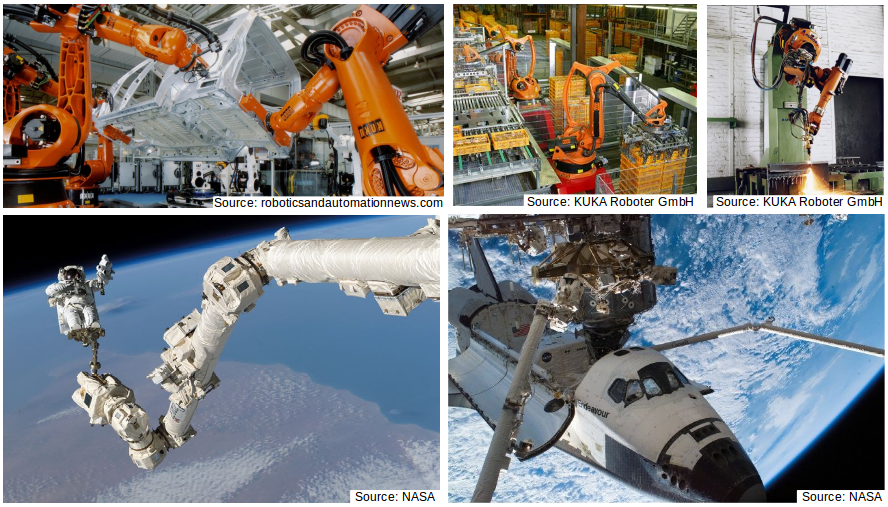Fig. 1.2  Robotic serial manipulators can be found in almost every industry

Robotic manipulators have become ubiquitous in almost every industry; from food, beverage, shipping and packaging to manufacturing, foundry and space:

• Palletizing food in a bakery
• Precision painting of automobiles and aircrafts
• Targeting products to their packaging stations in a warehouse
• Cutting steel and manufacturing of steel bridges
• Spot Welding and automating foundry processes
• Assisting in the deployment of satellites
• Docking and berthing of spacecrafts to the ISS
• Assisting in the mobility of astronauts during EVA

All of these jobs require the same core capability, namely, that of the robotic arm's end-effector to reach specific 3D coordinates within its workspace so that it can interact with the environment at that location, as this project aims to demonstrate.

### 2. Environment Setup

The project uses ROS Kinetic Kame running on Ubuntu 16.04 LTS (Xenial Xerus).

The following tools are used for simulation and motion planning:

• Gazebo: a physics based 3D simulator extensively used in the robotics world
• RViz: a 3D visualizer for sensor data analysis, and robot state visualization
• MoveIt!: a ROS based software framework for motion planning, kinematics and robot control

Once ROS is installed, we can proceed with the environment setup for the project:

##### Verify Project Tools

1. Verify the version of gazebo installed with ROS

```\$ gazebo --version
```

2. If the installed gazebo version is not 7.7.0+, update it as follows

```\$ sudo sh -c 'echo "deb http://packages.osrfoundation.org/gazebo/ubuntu-stable `lsb_release -cs` main" > /etc/apt/sources.list.d/gazebo-stable.list'
\$ wget http://packages.osrfoundation.org/gazebo.key -O - | sudo apt-key add -
\$ sudo apt-get update
\$ sudo apt-get install gazebo7
```
##### Create ROS Workspace

3. Create a catkin workspace if haven't already

```\$ mkdir -p ~/catkin_ws/src
\$ cd ~/catkin_ws/
\$ catkin_init_workspace
\$ ls -l
```

Notice that a symbolic link (CMakeLists.txt) has been created to `/opt/ros/kinetic/share/catkin/cmake/toplevel.cmake`

4. Clone or download project repository into the src directory of the catkin workspace

```cd ~/catkin_ws/src
\$ git clone https://github.com/Salman-H/pick-place-robot
```

5. Install missing dependencies if any

```\$ cd ~/catkin_ws
\$ rosdep install --from-paths src --ignore-src --rosdistro=kinetic -y
```

6. Change the permissions of script files to turn them executable

```\$ cd ~/catkin_ws/src/pick-place-robot/kuka_arm/scripts
\$ sudo chmod u+x target_spawn.py
\$ sudo chmod u+x IK_server.py
\$ sudo chmod u+x safe_spawner.sh
```

7. Build the project

```\$ cd ~/catkin_ws
\$ catkin_make
```

8. Open .bashrc file found in the home directory and add the following commands at the end

```# Inform Gazebo (sim software) where to look for project custom 3D models
export GAZEBO_MODEL_PATH=~/catkin_ws/src/pick-place-robot/kuka_arm/models

# Auto-source setup.bash since the pick and place simulator spins up different nodes in separate terminals
source ~/catkin_ws/devel/setup.bash
```

9. Save the .bashrc file and open a new terminal for changes to take effect

##### Test Simulator

The simulator environment can be tested by launching the project in demo mode.

10. Open `inverse_kinematics.launch` file under `/pick-and-place/kuka_arm/launch/` and set the demo flag to "true"

In addition, the spawn location of the target object can be modified if desired. To do this, modify the spawn_location argument in `target_description.launch`under `/pick-and-place/kuka_arm/launch/` where 0-9 are valid values for spawn_location with 0 being random mode.

11. Launch project by calling the safe_spawner shell script in a fresh terminal

```\$ cd ~/catkin_ws/src/pick-place-robot/kuka_arm/scripts
\$ ./safe_spawner.sh
```

Note: If Gazebo and RViz do not launch within a couple of seconds, close all processes started by this shell script by entering `Ctrl+C` in each of the sprung up terminals. Then rerun the safe_spawner script.

Once Gazebo and RViz are up and running, ensure the following can be seen in the gazebo world:

• Robot
• Shelf
• Blue cylindrical target in one of the shelves
• Dropbox right next to the robot

12. Run the `IK_server` ROS node from a new terminal window

```\$ cd ~/catkin_ws/src/pick-place-robot/kuka_arm/scripts
\$ rosrun kuka_arm IK_server.py
```

13. Arrange Gazebo and RViz windows side-by-side and click on Next button on left side of RViz to proceed between states.

The status message in RViz changes as the different stages of simulation are traversed with the Next button. Actuation is observed in the Gazebo window.

### 3. Theoretical Background

The following theoretical concepts are used in this project:

• Generalized Coordinates and Degrees of Freedom
• Common industrial serial manipulators and their workspace
• Rotation matrices and composition of rotations
• Euler angles and Euler theorem
• Homogeneous transforms
• Denavit–Hartenberg parameters
• Forward and Inverse Kinematics

#### 3.1 Serial Manipulators

Serial manipulators are robots composed of an assembly of links connected by joints (a Kinematic Chain), and the most common types of robots in industry.

##### Generalized Coordinates

Generalized coordinates are parameters that are used to uniquely describe the instantaneous dynamical configuration of a rigid multi-body system relative to some reference configuration. In the robotics of serial manipulators, they are used to define the configuration space or joint space, which refers to the set of all possible configurations a manipulator may have.

##### Degrees of Freedom

The degree of freedom (DOF) of a rigid body or mechanical system is the number of independent parameters or coordinates that fully define its configuration in free space.

Common DOFs:

• 6: coordinates required to fully describe the configuration of a rigid body in 3D free space
• 12: coordinates required to fully describe simultaneously the configuration of two separate rigid bodies in 3D free space
• 7: coordinates required to fully describe the configuration of two rigid bodies in 3D free space connected by a joint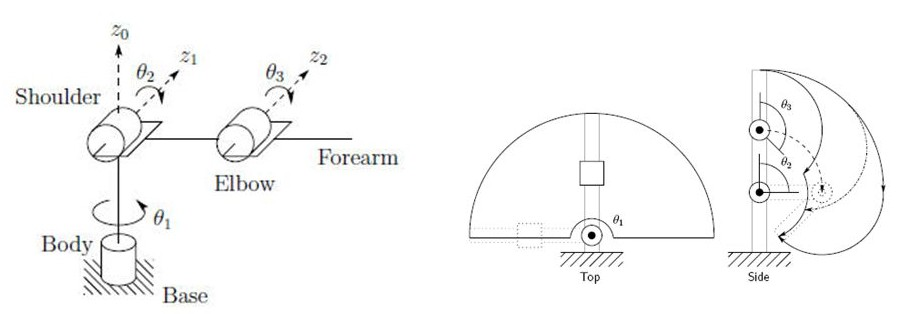Fig. 3.1  Geometry of a 3-DOF anthropomorphic robot
[Source: Narong Aphiratsakun. AIT]

The serial manipulator shown in figure 3.1 has n=3 joints: each a revolute with 1-DOF. Each joint connects with two links, making the total number of links, n+1 = 4, including the fixed base link.

Therefore, the total number of DOF for any serial manipulator with three 1-DOF joints is:

Note: The DOF of a serial manipulator with only revolute and/or prismatic joints is always equal to the number of its joints, except when both ends of the manipulator are fixed (closed chain linkage).

##### Workspace

The workspace of a robotic manipulator is defined as the set of points that can be reached by its end-effector . In other words, it is simply the 3D space in which the robot mechanism works.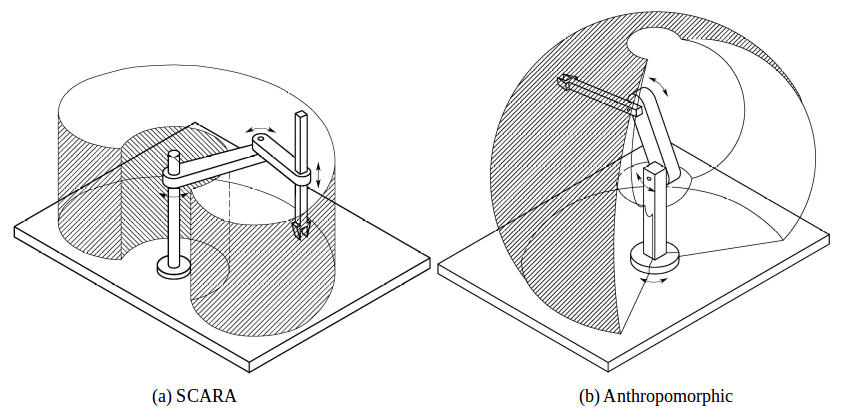Fig. 3.2   Workspaces of 3-DOF SCARA and anthropomorphic manipulators
[Source: Federica.EU]

Figure 3.2 shows two types of serial manipulators, SCARA and Anthropomorphic with their associated workspaces. Figure 3.1 also shows the workspace of the 3-DOF manipulator from a top and side perspective.

It is important to note that no kinematic solution exists for the manipulator's configuration or joint space for any desired end-effector position outside of the workspace.

##### Spherical Wrist

A spherical wrist of a robotic manipulator is designed by arranging its last three revolute joints such that their axes of rotations intersect at a common point, referred to as the wrist center.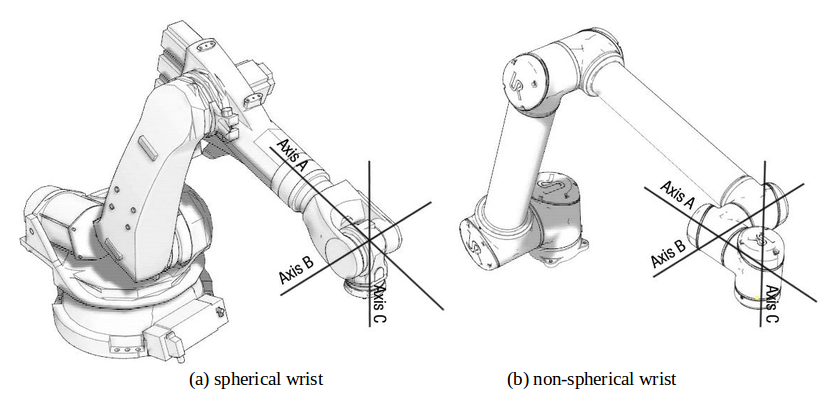Fig. 3.3  Difference between a spherical and non-spherical wrist
[Source: Khaled Elashry, ResearchGate]

Figure 3.3 shows the difference between a spherical and non-spherical wrist. In 3.3 (a), joint axes of rotations A, B, C all intersect at the wrist center, whereas, in 3.3(b), the wrist center is non-existent. Physically speaking, a six DOF serial manipulator like the one in figure 3.3 would use the first three joints to control the position of the wrist center while the last three joints (spherical wrist) would orient the end effector as needed, as in a human arm.

The spherical wrist is an important design characteristic in anthropomorphic manipulators which simplifies their kinematic analysis, as demonstrated in section 5.

#### 3.2 Rotation of Coordinate Frames

Rotation matrices are a means of expressing a vector in one coordinate frame in terms of some other coordinate frame.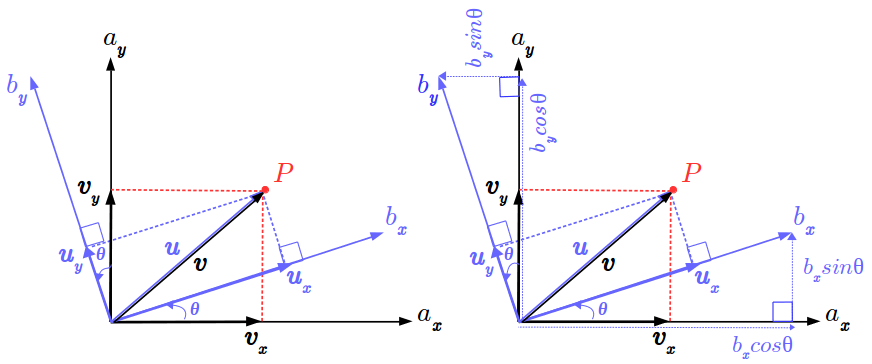Fig. 3.4  A 2D geometric rotation between coordinate frames A and B

In figure 3.2, Point P is expressed with vector u relative to coordinate frame B. The objective is to express point P with vector v relative to coordinate frame A. The basis vectors of v, vx and vy can be expressed in terms of the basis vectors of u, ux and uy as follows:

where unit vectors of frame A, ax and ay are expressed in terms of unit vectors of frame B, bx and by as follows:

Substituting (2) in (1) and solving for the dot products yields the following equation:

where the first term on the right-hand side is the 2D Rotation Matrix, denoted in this case as abR. Any point on coordinate frame B multiplied by abR will project it onto frame A. In other words, to express a vector u on some frame B as a vector v on a different frame A, u is multiplied by the rotation matrix with angle theta by which frame A is rotated from fram B. Also worth noting is that the rotation from A to B is equal to the transpose of the rotation of B to A.

#### 3.3 Euler Angles

Euler angles are a system to describe a sequence or a composition of rotations. According to Euler's Rotation Theorem, the orientation of any rigid body w.r.t. some fixed reference frame can always be described by three elementary rotations in a given sequence as shown in figure 3.3.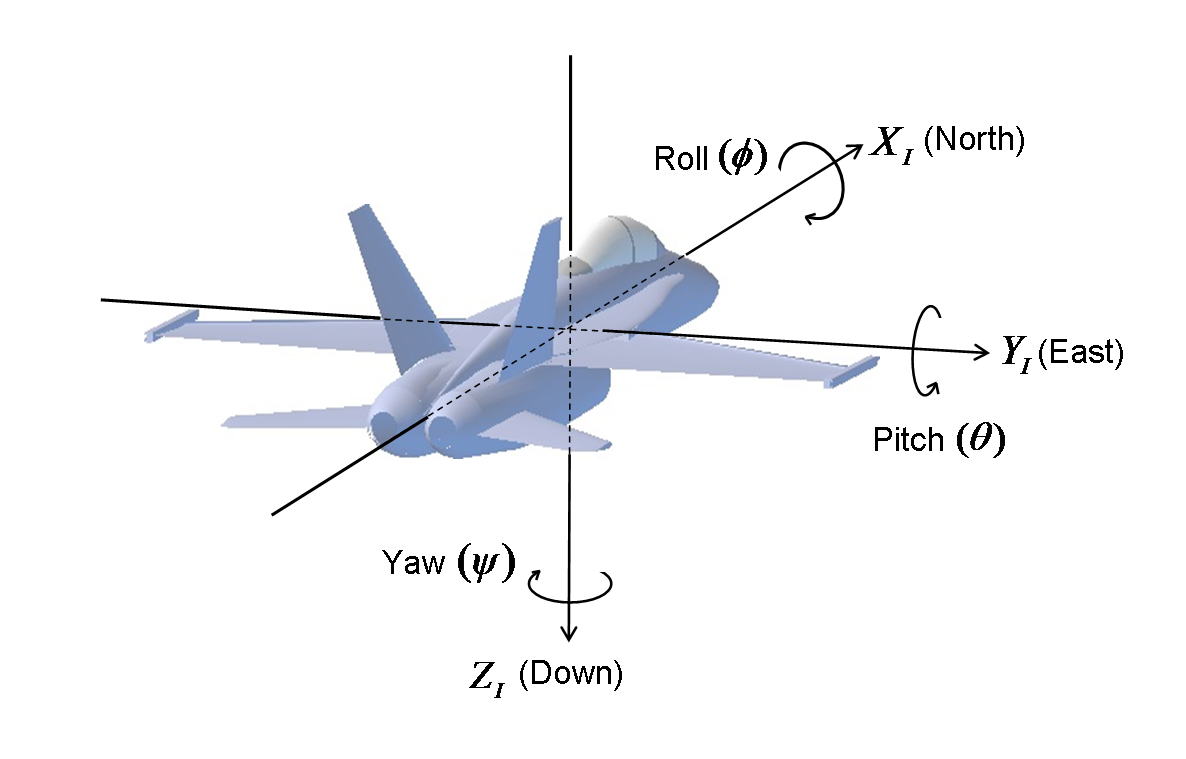Fig. 3.5  Defining Euler angles from a sequence of rotations
[Source: CHRobotics]

Conventionally, the movements about the three axes of rotations and their associated angles are described by the 3D rotation matrices in figure 3.4.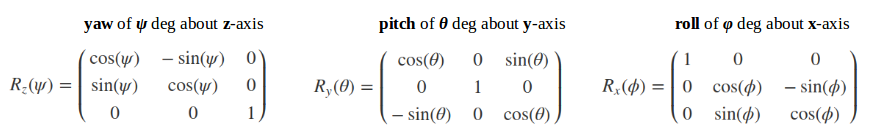Fig. 3.6  3D counter-clockwise rotation matrices describing yaw, pitch and roll

Euler angles are characterized by the following properties:

• Tait-Bryan vs. Classic
• Rotation Order
• Intrinsic (body fixed) vs. Extrinsic (fixed axes) rotations

Intrinsic or body-fixed rotations are performed about the coordinate system as rotated by the previous rotation. The rotation sequence changes the axis orientation after each elemental rotation while the body remains fixed.

In an intrinsic sequence of rotations, such as, a Z-Y-X convention of a yaw, followed by a pitch, followed by a roll, subsequent elemental rotations are post-multiplied.

Extrinsic or fixed-axis rotations are performed about the fixed world reference frame. The original coordinate frame remains motionless while the body changes orientation.

In an extrinsic sequence of rotations, such as, a Z-Y-X convention of a yaw, followed by a pitch, followed by a roll, subsequent elemental rotations are pre-multiplied.

Note: An extrinsic rotation sequence of A, B, C = an intrinsic rotation sequence of C, B, A.

Euler angles, normally in the Tait–Bryan, Z-X-Y convention, are also used in robotics for describing the degrees of freedom of a spherical wrist of a robotic manipulator.

Of particular importance is a phenomenon associated with Euler angles known as a Gimbal Lock which occurs when there is a loss of one degree of freedom as a result of the axes of two of the three gimbals driven into a parrallel configuration.

#### 3.4 Homogeneous Transforms

In the case where a reference frame is both simultaneously rotated and translated (transformed) with respect to some other reference frame, a homogeneous transform matrix describes the transformation.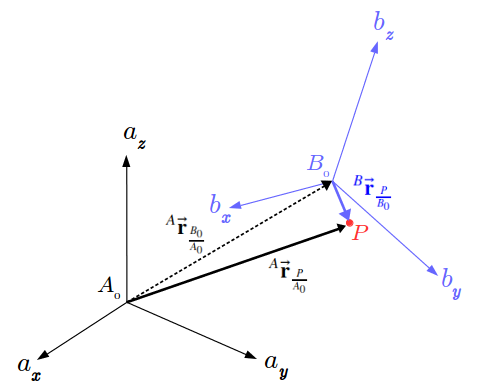Fig. 3.7  Rotation and Translation of frame B relative to frame A

In figure 3.7, point P is expressed w.r.t. frame B and the objective is to express it w.r.t. frame A. To do so would require projecting or superimposing frame B onto frame A i.e. first rotating frame B to orient it with frame A and then translating it such that the centers B0 and A0 of both frames are aligned.

The relationship between the three vectors in figure 3.7 is shown in equation (1). The desired vector to point P from A0 is the sum of the vector to point P from B0, rotated to frame A, and the translation vector to B0 w.r.t A0. Equations (2) and (3) are the matrix-forms of equation (1) so that it can be rendered in software with linear algebra libraries.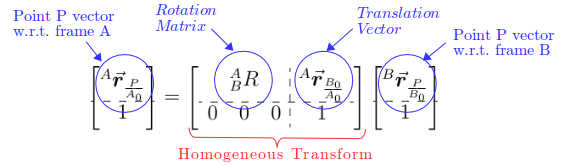Fig. 3.8  Anatomy of the homogeneous transform relationship

Figure 3.8 describes the components of equation (2). The desired vector to point P (w.r.t. to A0) is obtained by multiplying the given vector to point P (w.r.t. B0) by the homogeneous transform matrix, composed of the block Rotation matrix projecting B onto A and the block translation vector to B w.r.t A0.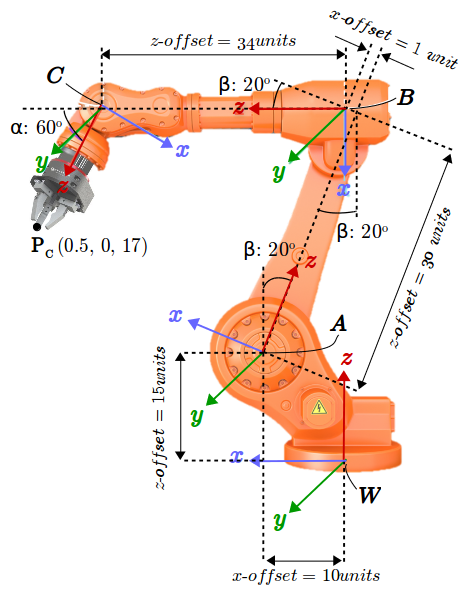Fig. 3.9  Transformation between adjacent revolute joint frames

As shown in figure 3.9, the position of the end-effector is known w.r.t. its coordinate reference frame C. The objective is to express it w.r.t. the fixed world coordinate reference frame W. This is because the positions of all objects of interest in the manipulator's environment are expressed w.r.t. the world reference frame. In other worlds, both, the end-effector, and the objects it interacts with need to be defined on the same coordinate reference frame.

Point P relative to frame W can be found by successively applying equation (4) between adjacent joints:

The above process can be summarized in terms of equation (1) with WCT being the desired composite homogeneous transform that projects frame C onto frame W.

#### 3.5 Denavit–Hartenberg parameters

Before the homogeneous transforms between adjacent links can be computed, the coordinate frames of the joint links on which the transforms are applied must be defined. The Denavit–Hartenberg (DH) parameters are four parameters describing the rotations and translations between adjacent links. The definition of these parameters constitutes a convention for assigning coordinate reference frames to the links of a robotic manipulator. Figure 3.8 shows the so-called modified convention of DH parameters as defined by [Craig, JJ. (2005)].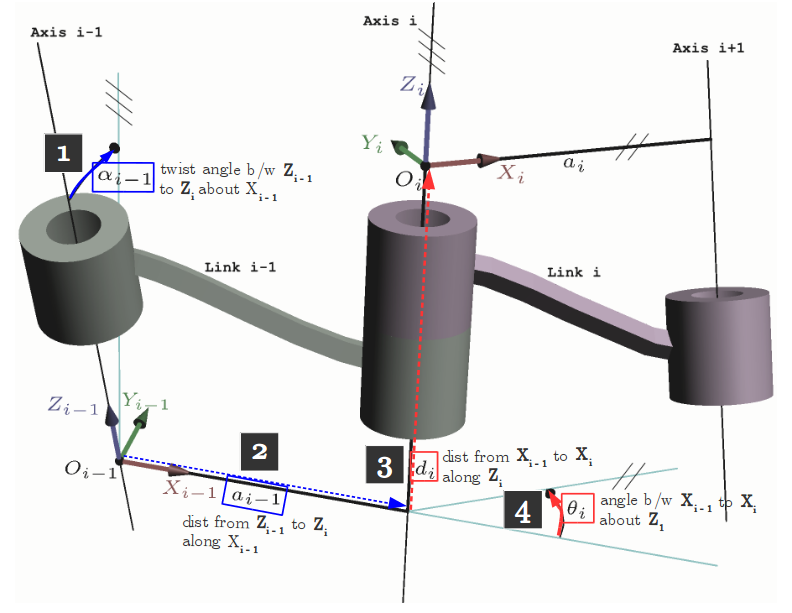Fig. 3.8  The four parameters of the Modified DH convention
[Source: Modified from Wikipedia Commons]

The parameters are defined as follows:

• αi-1: twist angle between the z-axes of links i-1 and i (measured about xi-1 in a right-hand sense)
• ɑi-1: link distance between the z-axes of links i-1 and i (measured xi-1)
• di: link offset signed distance between the x-axes of links i-1 and i (measured along zi)
• θi: joint angle between the x-axes of links i-1 and i (measured about zi in a right-hand sense)

Note:

• The origin of a frame i is defined by the intersection of xi and zi
• The x-axes define the common normals between zi-1 and zi

Recall that to compute the position of the end-effector w.r.t. the base or world reference frame, transforms between adjacent links are composed as follows:

where the base frame is denoted by 0 and the end-effector's frame denoted by N. Thus, 0NT defines the homogeneous transformation that projects frame N onto frame 0. More specifically, a single transform between links i-1 and i is given by

and is made up up of two rotations R of magnitudes α and θ, and two displacements D of magnitudes ɑ and d.

The parameter assignment process for open kinematic chains with n degrees of freedom (i.e., joints) is summarized as:

1. Label all joints from {1, 2, … , n}.
2. Label all links from {0, 1, …, n} starting with the fixed base link as 0.
3. Draw lines through all joints, defining the joint axes.
4. Assign the Z-axis of each frame to point along its joint axis.
5. Identify the common normal between each frame Zi-1 and Zi
6. The endpoints of intermediate links (i.e., not the base link or the end effector) are associated with two joint axes, {i} and {i+1}. For i from 1 to n-1, assign the Xi to be ...
1. For skew axes, along the normal between Zi and Zi+1 and pointing from {i} to {i+1}.
2. For intersecting axes, normal to the plane containing Zi and Zi+1.
3. For parallel or coincident axes, the assignment is arbitrary; look for ways to make other DH parameters equal to zero.
7. For the base link, always choose frame {0} to be coincident with frame {1} when the first joint variable (θ1 ​​ or d1) is equal to zero. This will guarantee that α0 = a0 = 0, and, if joint 1 is a revolute, d1 = 0. If joint 1 is prismatic, then θ1 = 0.
8. For the end effector frame, if joint n is revolute, choose Xn to be in the direction of Xn−1 ​​ when θn​ = 0 and the origin of frame {n} such that dn = 0.

Special cases involving the Zi-1 and Zi axes:

• collinear lines: alpha = 0 and a = 0
• parallel lines: alpha = 0 and a ≠ 0
• intersecting lines: alpha ≠ 0 and a = 0
• If the common normal intersects Zi at the origin of frame i, then d​i is zero.

Once the frame assignments are made, the DH parameters are typically presented in tabular form (below). Each row in the table corresponds to the homogeneous transform from frame {i} to frame {i+1}.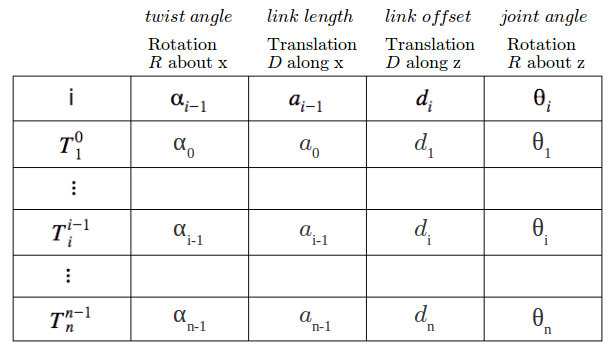Table 3.1  The four parameters of the Modified DH convention

#### 3.6 Forward and Inverse Kinematics

Forward Kinematics is the process of computing a manipulator's end-effector position in Cartesian coordinates from its given joint angles. This can be achieved by a composition of homogeneous transformations that map the base frame onto the end-effector's frame, taking as input the joint angles. The end-effector's coordinates can then be extracted from the resulting composite transform matrix.

The relationship between Forward and Inverse Kinematics is depicted in figure 3.9,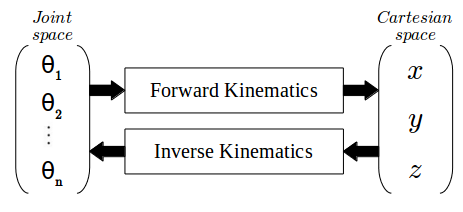Fig. 3.9  Relationship between Forward and Inverse Kinematics

Inverse Kinematics is the reverse process where the EE position is known and a set of joint angles that would result in that position need to be determined. This is a more complicated process than FK as multiple solutions can exist for the same EE position. However, no joint angle solutions exist for any EE position outside the manipulator's workspace. There are two main approaches to solve the IK problem: numerical and analytical. The later approach is used in this project.

### 4. Design Requirements

The scope of the design is limited to a single pick-and-place cycle that consists of the following steps:

1. Movement of EE towards the target object
2. Grasping/picking the target object
3. Movement towards the drop-site
4. Dropping/placing the object at the drop-site

Figure 4.1 shows these steps in Gazebo.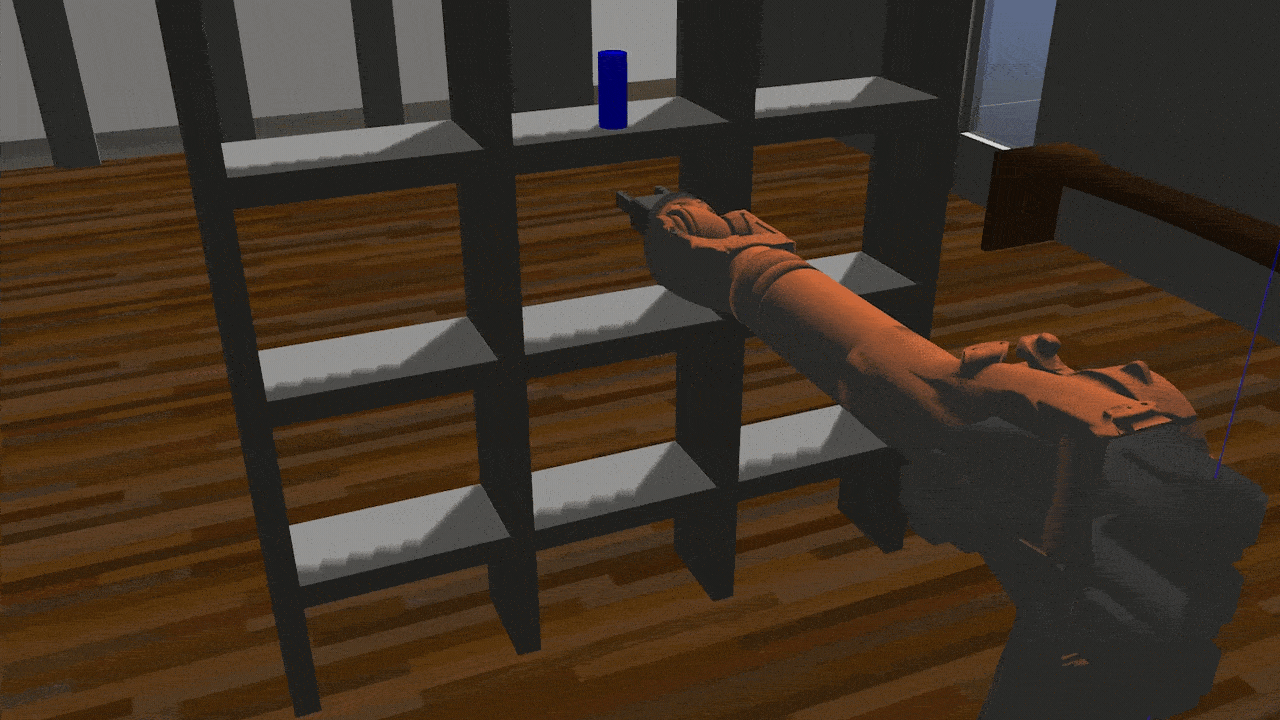Fig 4.1  A single pick-and-place cycle
[Source: Gazebo]

The primary metrics of interest are:

• Percentage of success in a total of 10 pick-and-place cycles
• Error in the calculated EE position trajectory (via FK) compared to the EE position received in the IK service request
• Time taken to execute a complete pick-and-place cycle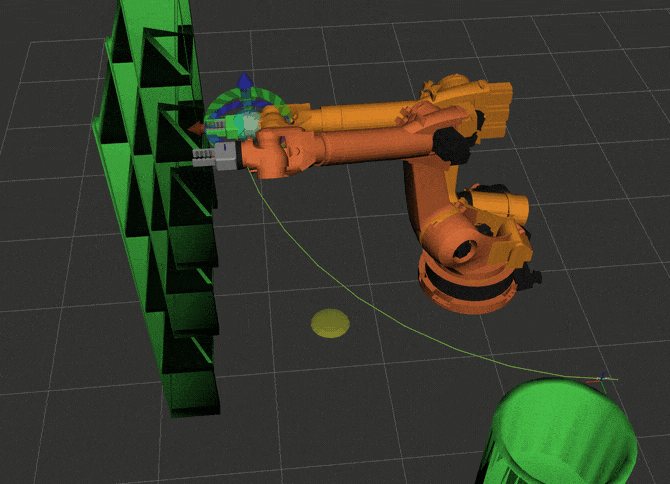Fig 4.2  Planned EE trajectory to drop-off location
[Source: RViz, MoveIt!]

Table 4.1 shows the criteria on which the project is evaluated,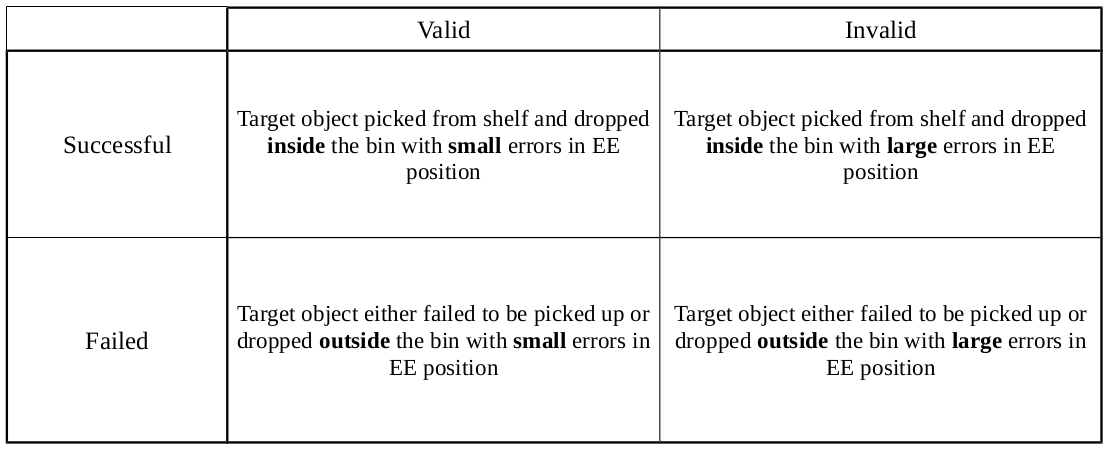Table 4.1  Project evaluation criteria

The minimum criteria is to achieve a success rate of at least 80% with an EE trajectory error not greater than 0.5.

### 5. Design Implementation

In order to perform a single pick-and-place operation, joint angles corresponding to the given locations of the target object and drop-off site need to be determined. Towards this goal, an Inverse Kinematic analysis is performed and implemented in software using ROS and Python.

#### 5.1 Kinematic Analysis

##### DH Parameters

Figure 5.1 (a) shows the Kuka KR210 serial manipulator and (b) shows its zero configuration schematic indicating its DH parameters. In the zero configuration, all joint angles are assumed to be zero.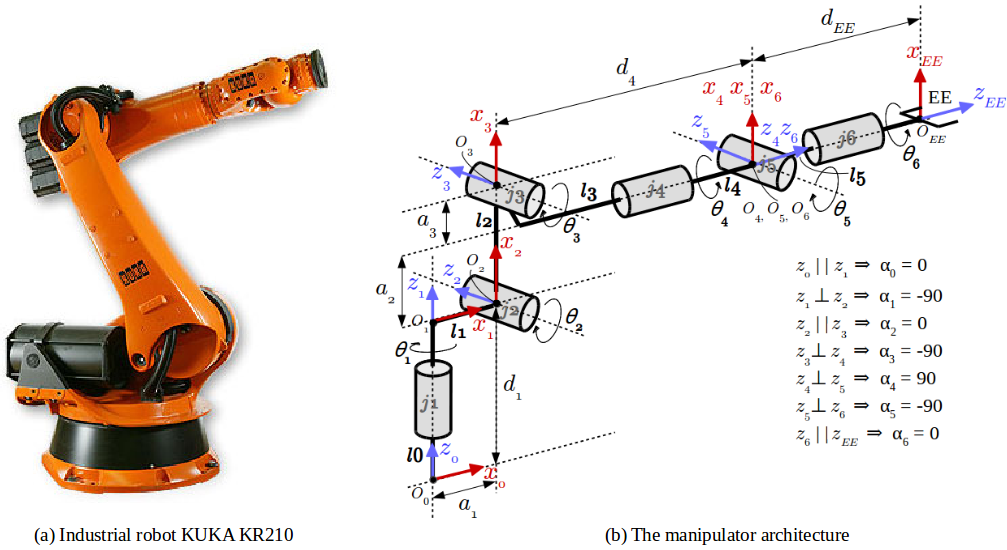Fig. 5.1  The KUKA KR210 6-DOF robotic manipulator and its schematic architecture
[Source: (a) KUKA Roboter GmbH, (b) Salman Hashmi, BSD License]

Note that, for joint 2, there is a constant -90 degree offset between x1 and x2.

The following steps are performed to construct the KR210 schematic and derive its DH Table:

1. Label joints from 1 to n = 6
2. Label each link from 0 to n = 6
3. Define z-axes as the joint axes (joints 2, 3, and 5 are all parallel while joints 4 and 6 are coincident)
4. Define x-axes as the common normals
5. Define reference frame origins for each joint
6. Define the x-axes as the common normals between zi-1 and zi
7. Define the origin of frame {i} as the intersection of xi with zi
8. Add a fixed frame rigidly attached to link 6 for the gripper or EE (Note: the EE reference frame OEE differs from the link 6 reference frame of O4, O5, O6 only by a translation along z6)
9. Label all non-zero DH parameters

The last step is implemented using table 5.1 which is constructed from the KR210 URDF file, `kr210.urdf.xacro`.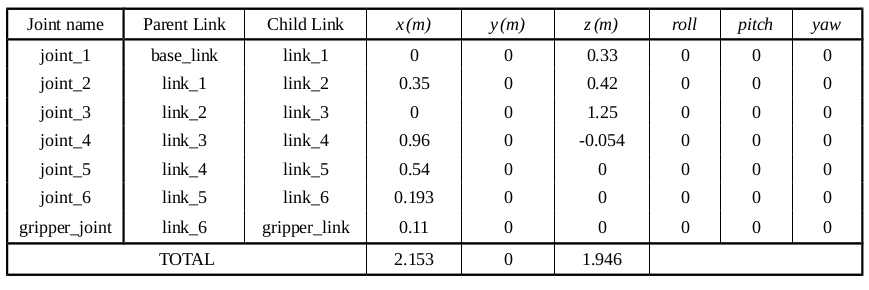Table 5.1  Location of joint {i} relative to its parent joint {i-1} from the KR210 URDF file

Note the following concerning the URDF file and table 5.1,

• RViz does not directly show joint reference frames but instead shows link reference frames
• In th URDF file, each joint is defined relative to the center (not origin) of its parent joint e.g. row 2 shows the location of joint 2 relative to the center (not origin) of joint 1
• The base_link in Table 5.1 is depicted as link0 (l0) in figure 5.1 (b)

The DH Table is then derived from figure 5.1 (b) and table 5.1,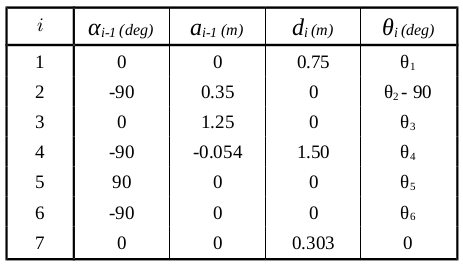Table 5.2  Modified DH Table of the KR210

##### Inverse Kinematic Solution Approach

An analytical or closed-form approach is used to perform inverse kinematics. This approach has two advantages:

1. Much faster to solve compare to a numerical approach
2. Easier to develop rules for which solution is appropriate

Additionally, The type of robotic manipulator used (anthropomorphic) meets the conditions for applicability of this approach:

1. Axes of rotations of 3 adjacent joints should intersect at a single point (satisfied by a spherical wrist design)
2. Axes of rotations of 3 adjacent joints are parallel (special case of 1. since parallel lines intersect at infinity)

The 4 x 4 homogeneous transform between adjacent links from section 3 is shown here again for clarity:

It is noted that 12 simultaneous nonlinear equations would have to be solved, one for each term in the first 3 rows of the overall homogeneous transformation between the base link and the end-effector. Therefore, to simplify the solution, The spherical wrist design is exploited to kinematically decouple the position and orientation of the end-effector such that the original problem is reduced to two simpler problems that can be solved independently:

1. Find position (Cartesian coordinates) of WC and geometrically compute joint angles 1, 2, 3 that physically control it
2. Find the composition of x-y-z rotations (r, p, y) that orients EE and analytically solve its Euler angles: joint angles 4, 5, 6
##### Inverse Position Kinematics

As mentioned previously, joints 1, 2, and 3, control the position of the spherical wrist consisting of joints 4, 5, and 6. In the position part of Inverse Kinematics, joint angles 1, 2, and 3 are geometrically calculated from the position of the spherical wrist center (WC). This position is determined from the end-effector position (EE) using figure 5.2.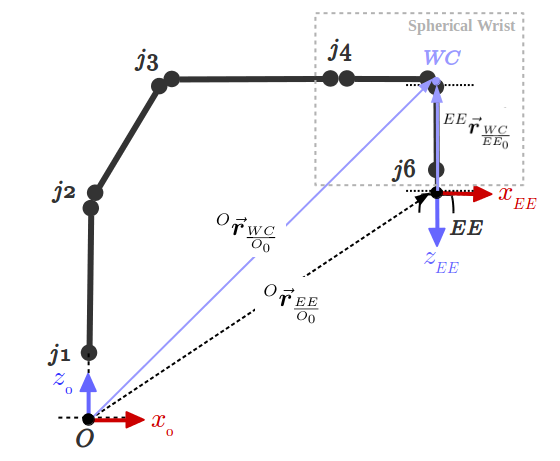Fig. 5.2  Finding location of WC relative to base frame O

The position vector of WC w.r.t. to EE (rWC/EEO) is a simple translation along zEE. The desired position vector of WC w.r.t. the base frame O can be found by transforming rWC/EEO onto the base frame O using a homogeneous transform consisting of Rotation matrix 0EER and a translation vector from O to EE,

where dEE is given by d7 in the DH Table 5.2, and the column 3 vector of the Rotation Matrix describes the z-axis of EE relative to base frame O.

Once the Cartesian coordinates of WC are known, θ1 and θ2 can be calculated using the Law of Cosines on an SSS triangle with edges at joints 2, 3 and 5, as shown in figure 5.3.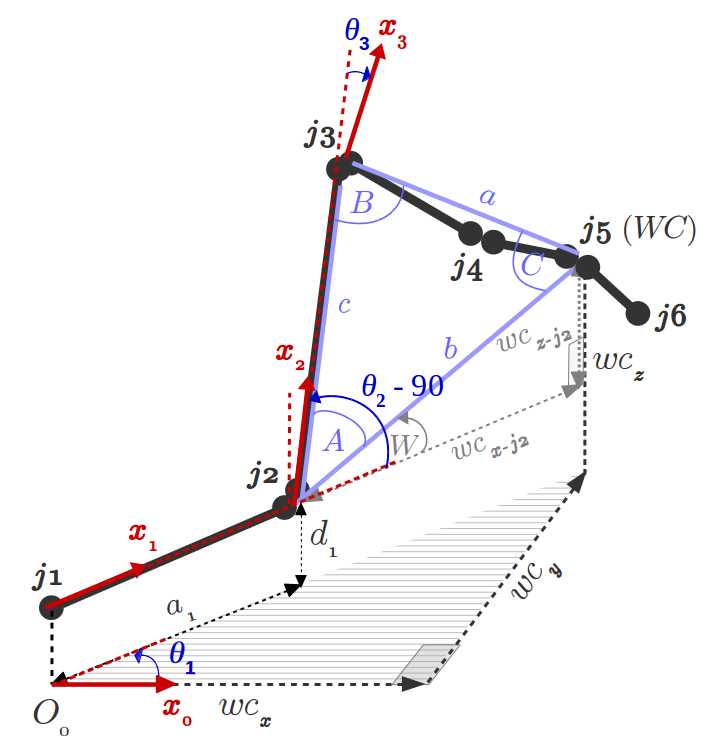Fig. 5.3  Calculation of θ1 and θ2 using an SSS triangle

Note: Joint angles are defined as rotations about z between adjacent x axes, e.g. joint angle θ3 is the angle between x2 and x3 about z3 (not shown).

θ1 is the joint-1 angle between x0 and x1 measured about z1. It is calculated using the x and y coordinates of WC relative to the base frame,

θ2 is the joint-2 angle between x1 and x2 measured about z2. Note that, for joint 2, there is a constant -90 degree offset between x1 and x2 as shown in figure 5.1 (b) and in DH Table 5.2 for i = 2,

where W is given by,

and A is determined by the Law of Cosines.

θ3 is the joint-3 angle between x2 and x3 measured about z3. Figure 5.4 (b) is used to calculate θ3 where 5.4 (a) is used as a comparison to help visualize the sag in the links between joints 3 and 5.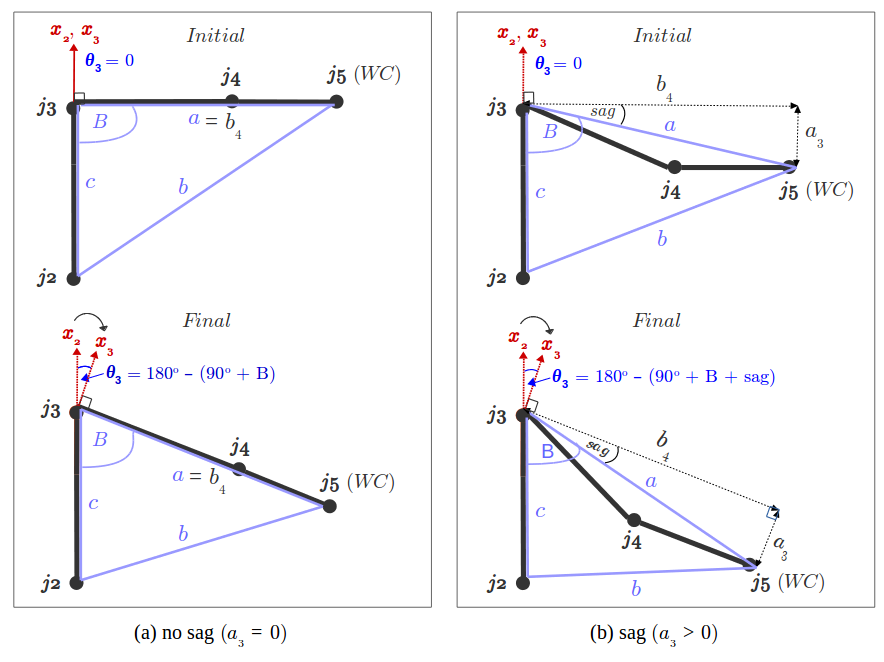Fig. 5.4  Calculation of θ3 and accounting for sag in the links between j3 and j5

As described in figure 5.4 (b) Final, θ3 is given by,

where the sag angle is,

and B is determined by the Law of Cosines.

##### Inverse Orientation Kinematics

Recall that joints 4, 5, and 6 constitute the spherical wrist design, where joint 5 is the wrist center (WC). In the orientation part of Inverse Kinematics, joint angles 4, 5, and 6 are analytically calculated from 36R; the composition of x-y-z rotations (roll, pitch, yaw) that orients the WC. Thus, joint angles 4, 5, and 6 are the Euler angles of this composition of rotations.

36R can be determined from 06R as follows,

where i-1iR is the composite rotation matrix from the homogeneous transform i-1iT,

and 03R is given by,

and since joint angles θ1, θ2, and θ3 have already been calculated, 03R is no longer a variable as θ1, θ2, and θ3 can simply be substituted in 01R, 12R, and 23R respectively, leaving θ4, θ5, and θ6 as the only variables in 36R.

Symbolically evaluating 36R in sympy yields,

Joint angles, θ4, θ5, and θ6 can then be analytically determined from 36R,

#### 5.2 Software Implementation

The primary code for the project is in the ROS node, `IK_server` in the `kuka_arm` ROS package, and the KR210 is operated in a ROS based simulator environment consisting of Gazebo, RViz and MoveIt!.

The `IK_server` node receives end-effector (gripper) poses from the KR210 simulator and performs Inverse Kinematics, providing a response to the simulator with calculated joint variable values (joint angles in this case). The IK analysis conducted in section 5.1 is implemented in the `IK_server` node using Sympy and Numpy libraries.

The following are selected notes of interest regarding this implementation:

• All python code conforms to PEP 8 wherever possible with occasional deviations to accommodate Sympy and Numpy matrix notations, and where it improves readability. Similarly, function docstrings are conformed to PEP 257 convention.

• The DH table for the KR210 is constructed from the `kr210.urdf.xacro` file in the `kuka_arm` ROS package.

• The gripper or EE orientations are converted to the desired Euler angles from the quaternions received in the IK service request using ROS geometry transformations module.

• URDF vs DH frame misalignment in gripper/EE pose is addressed by aligning the URDF and DH EE frames through a sequence of intrinsic rotations: 180 deg yaw and -90 deg pitch.

• In the Sympy implementation, the DH Table is substituted in individual transforms before composing the overall homogeneous transform between the base frame and the end-effector. This is because it takes considerable calculation time (in the order of several seconds) to symbolically simplify the product of six 4x4 transform matrices each consisting of trigonometric expressions. By substituting some of the values for constants and angles in these trigonometric expressions for each individual matrix, the overall composite homogeneous transform matrix is simplified much faster (down to the order of milliseconds).

• As described in figures 5.3 and 5.4, to account for sag in side_a of the SSS triangle (the line segment connecting joints 3 and 5 (WC)) caused by joint 4, first, length of side_a is recalculated, and second, the sag angle formed between y3-axis and side_a is calculated and accounted for in the calculation of `theta_3`.

• Once the composite Rotation matrix, `R3_6`, is symbolically evaluated using Sympy for calculating thetas 1, 2, 3, the `IK_server` node is reimplemented in Numpy to optimize speed:

• mpmath matrix and trig imports are replaced with numpy
• dh params (dict keys) are redefined as strings instead of sympy symbols
• `q` symbols are replaced with `thetas` to make joint variables consistent
• dh params are moved from global scope since joint variables are not immutable like sympy symbols
• sympy matrices are replaced with numpy matrices
• dh variable is added to function args and accessed with string keys
• `R0_3` is converted to a float array before passing in `numpy.lingalg.inv` to compute inverse
• Remove single and composite transforms (required for fk only)
• joint angles are updated in dh params dictionary after they are calculated
• To improve accuracy of theta 2 and 3, both the length of side_a (containing sag) and the sag angle are rounded-off to a high and consistent number of digits

### 6. Testing and Review

##### Testing

After implementing the `IK_server` ROS node, the pick-and-place operation can be tested by launching the project in test mode by setting the demo flag to "false" in `inverse_kinematics.launch` file under `/pick-and-place/kuka_arm/launch/`.

In addition, the spawn location of the target object can be modified if desired. To do this, modify the spawn_location argument in `target_description.launch`under `/pick-and-place/kuka_arm/launch/` where 0-9 are valid values for spawn_location with 0 being random mode.

The project is launched by calling the `safe_spawner` shell script in a fresh terminal

```\$ cd ~/catkin_ws/src/pick-place-robot/kuka_arm/scripts
\$ ./safe_spawner.sh
```

Note: If Gazebo and RViz do not launch within a couple of seconds, close all processes started by this shell script by entering `Ctrl+C` in each of the sprung up terminals. Then rerun the safe_spawner script.

Once Gazebo and RViz are up and running, and the following can be seen in the gazebo world:

• Robot
• Shelf
• Blue cylindrical target in one of the shelves
• Dropbox right next to the robot

The `IK_server` ROS node is run from a new terminal window as follows

```\$ cd ~/catkin_ws/src/pick-place-robot/kuka_arm/scripts
\$ rosrun kuka_arm IK_server.py
```

With Gazebo and RViz windows arranged side-by-side, the Next button on left side of RViz window can be clicked to proceed from one state to another, while the Continue button can be clicked to continuously run a complete pick-and-place cycle.

Warning: The terminal window from which the `safe_spawner` shell script is called needs to be monitored for a `Failed to call service calculate_ik` error, in which case, all running processes need to be killed and the `safe_spawner` script called again.

As shown in figure 6.1, the status message in RViz changes as the different stages of pick-and-place simulation are traversed. Actuation is observed in the Gazebo window.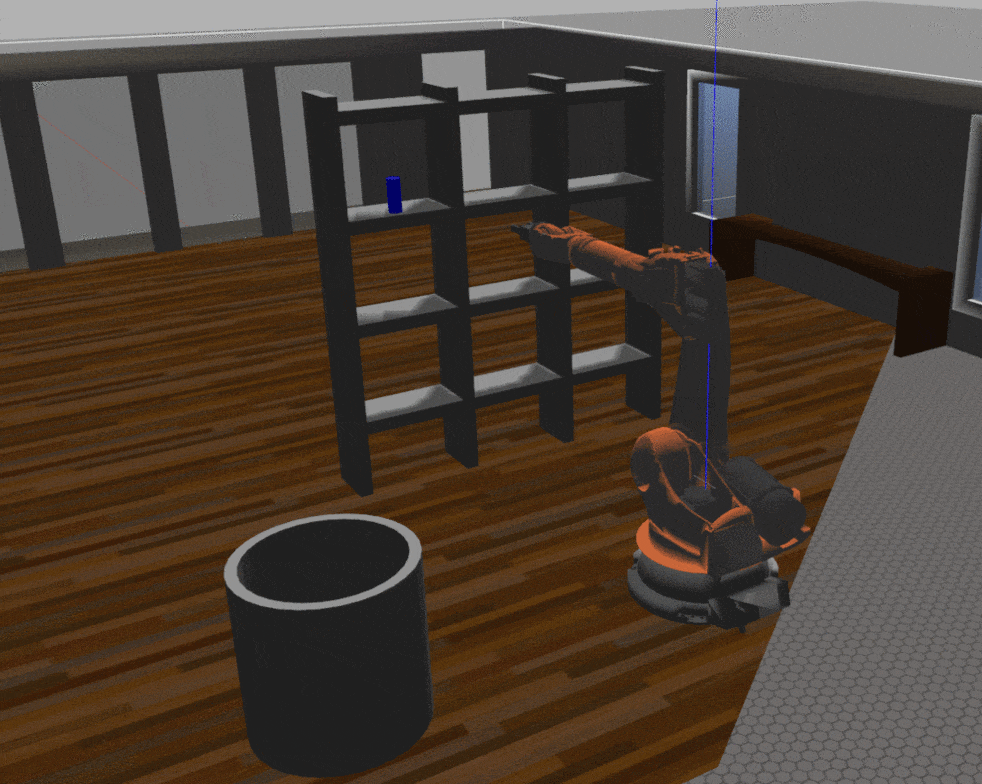Fig. 6.1  Steps followed in a single pick-and-place cycle
[Source: Gazebo, MoveIt!]

##### Review

The primary metric of interest is the error in the calculated EE trajectories. Since multiple joint angle values can lead to the same EE position, error in joint angle values is not a reliable indicator of whether the EE position is being calculated correctly. The only way to know conclusively is to substitute the joint angle values from IK into FK and compare the resulting EE position to the one received in the IK service request.

As defined in section 4, the three evaluation metrics are:

• Success Rate:    Percentage of success in a total of 10 pick-and-place cycles (success defined in Table 4.1)
• EE Error:            Error in the calculated EE position trajectory (via FK) compared to the one received in IK request
• Time Taken:       Average time taken for a total of 10 pick-and-place cycles

Figure 6.2 shows the KR210 execute a planned EE trajectory to the drop-off location with joint angles obtained from the IK implementation.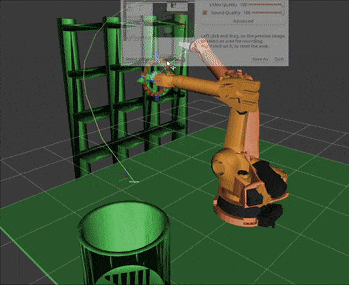Fig. 6.2  Executing a planned EE trajectory
[Source: MoveIt!]

A cursory visual inspection of the animation in figure 6.2 shows that the trajectory of received EE positions in the IK service request is being correctly followed by the six joints of the KR210. The same is observed in Figure 6.3 which comprehensively compares various requested EE trajectories with the followed EE trajectories and shows the overall EE position error for each.

Figure 6.3 (a) shows six EE position trajectories received by the `IK_server` ROS node in the IK service request; A, C, and E to the target object (not visible) and B, E, and F to the drop-off location. Figure 6.3 (b) compares these received EE positions (rec_ee in blue) to the ones obtained by FK (fk_ee in orange) from the six joint angles. Due to a very low resulting EE overall offset error (ee_error in magenta), the blue plot points of the received EE positions are hidden behind the orange plot points of the EE positions obtained from FK. A visual inspection also shows

##### Results

After a total of 10 runs in the simulator, the following results are achieved:

• Success Rate:       100%
• EE Error:               0.00000006
• Average Time:      51 sec
##### Improvements

In addition to the accuracy improvements made in the calculation of theta2 and theta3 in section 5.1, as well as a 350x time improvement with a Numpy implementation of the IK_server node, the following can offer further improvements in EE error and speeds,

• Ensuring precision of intermediate calculation results when solving for joint angles
• Use of quaternions instead of Euler angles
• Solving the SSS triangle with a slightly faster Law of Cosines alternative:
1. Using The Law of Cosines to calculate the largest angle
2. Using The Law of Sines to find another angle
3. Using angles of a triangle add to 180° to find the last angle.

### References

Get A Weekly Email With Trending Projects For These Topics
No Spam. Unsubscribe easily at any time.
python (48,505
c-plus-plus (17,009
object-detection (417
ros (227
gazebo (31
motion-planning (31